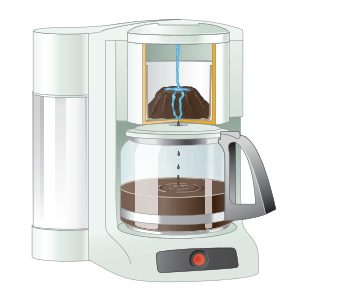×
Get Full Access to Chemistry: The Central Science - 14 Edition - Chapter 1 - Problem 1.5
Get Full Access to Chemistry: The Central Science - 14 Edition - Chapter 1 - Problem 1.5

×

# ?Is the separation method used in brewing a cup of coffee best described as distillation, filtration, or chromatography? [Section 1.3]ISBN: 9780134414232 1274

## Solution for problem 1.5 Chapter 1

Chemistry: The Central Science | 14th Edition

• Textbook Solutions
• 2901 Step-by-step solutions solved by professors and subject experts
• Get 24/7 help from StudySoup virtual teaching assistantsChemistry: The Central Science | 14th Edition

4 5 1 267 Reviews
11
2
Problem 1.5

Is the separation method used in brewing a cup of coffee best described as distillation, filtration, or chromatography? [Section 1.3]Step-by-Step Solution:

Step 1 of 5) Many properties of matter are quantitative, that is, associated with numbers. When a number represents a measured quantity, the units of that quantity must be specified. To say that the length of a pencil is 17.5 is meaningless. Expressing the number with its units, 17.5 centimeters (cm), properly specifies the length. The units used for scientific measurements are those of the metric system. The metric system, developed in France during the late eighteenth century, is used as the system of measurement in most countries. The United States has traditionally used the English system, although use of the metric system has become more common In 1960, an international agreement was reached specifying a particular choice of metric units for use in scientific measurements. These preferred units are called SI units, after the French Système International d’Unités. This system has seven base units from which all other units are derived (Table 1.3). In this chapter we will consider the base units for length, mass, and temperature. There is nothing mysterious about how scientists work. The first idea to keep in mind is that scientific knowledge is gained through observations of the natural world. A principal aim of the scientist is to organize these observations by identifying patterns and regularity, making measurements, and associating one set of observations with another. The next step is to ask why nature behaves in the manner we observe. To answer this question, the scientist constructs a model, known as a hypothesis, to explain the observations. Initially, the hypoth

Step 2 of 1

##### ISBN: 9780134414232

This full solution covers the following key subjects: . This expansive textbook survival guide covers 29 chapters, and 2820 solutions. This textbook survival guide was created for the textbook: Chemistry: The Central Science, edition: 14. The answer to “?Is the separation method used in brewing a cup of coffee best described as distillation, filtration, or chromatography? [Section 1.3]” is broken down into a number of easy to follow steps, and 20 words. Since the solution to 1.5 from 1 chapter was answered, more than 217 students have viewed the full step-by-step answer. Chemistry: The Central Science was written by and is associated to the ISBN: 9780134414232. The full step-by-step solution to problem: 1.5 from chapter: 1 was answered by , our top Chemistry solution expert on 10/03/18, 06:29PM.

## Discover and learn what students are asking

Calculus: Early Transcendental Functions : Integration Techniques, LHpitals Rule, and Improper Integrals
?In Exercises 49-56, find the indefinite integral using any method. $$\int \theta \sin \theta \cos \theta d \theta$$

Statistics: Informed Decisions Using Data : Properties of the Normal Distribution
?In Problems 25–28, the graph of a normal curve is given. Use the graph to identify the values of ? and ?.

Statistics: Informed Decisions Using Data : Estimating a Population Standard Deviation
?True or False: The shape of the chi-square distribution depends on its degrees of freedom.

Statistics: Informed Decisions Using Data : Inference about Two Means: Independent Samples
?Explain why using the smaller of n1 - 1 or n2 - 1 degrees of freedom to determine the critical t instead of Formula (2) is conservative.

Unlock Textbook Solution